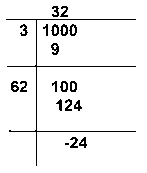# Find the least number of four digits

Question:

Find the least number of four digits that is a perfect square.

Solution:

Since, the greatest number of three digits is 1000 .

Now, find the square root of 1000 by long division method.∴The least number of four digits that is a perfect square = 1000 + 24

= 1024